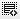How to calculate Dominos salary and interest expense?0
How is this calculated?
In the Dominos Pizza Income Statement (from the video), the Salary expense grows by how much % from 2008 to 2009
3/3
0.4 - Correct
0.3
0.31
0.23
From the Dominos Pizza Income Statement (in the video), how much additional interest expense does Dominos pay in 2009 compared to 2008?
3/3
Rs. 40.84 million - Correct
Rs. 42.48 million
Rs. 38.98 million
Rs. 48.78 million0

In page 12 of this document is the income statement - http://www.sebi.gov.in/dp/jubilantdraft.pdf
The interest expenses are termed under "finance charges". 2008 interest expense is 58.11 and 2009 interest expense is 98.95. If you subtract them you will get the correct answer.0

sorry, i mistakenly copied two questions.
i wanted to ask
How is this calculated?
In the Dominos Pizza Income Statement (from the video), the Salary expense grows by how much % from 2008 to 2009
3/3
0.4 - Correct
0.3
0.31
0.23

Click on thisicon to add code snippet.